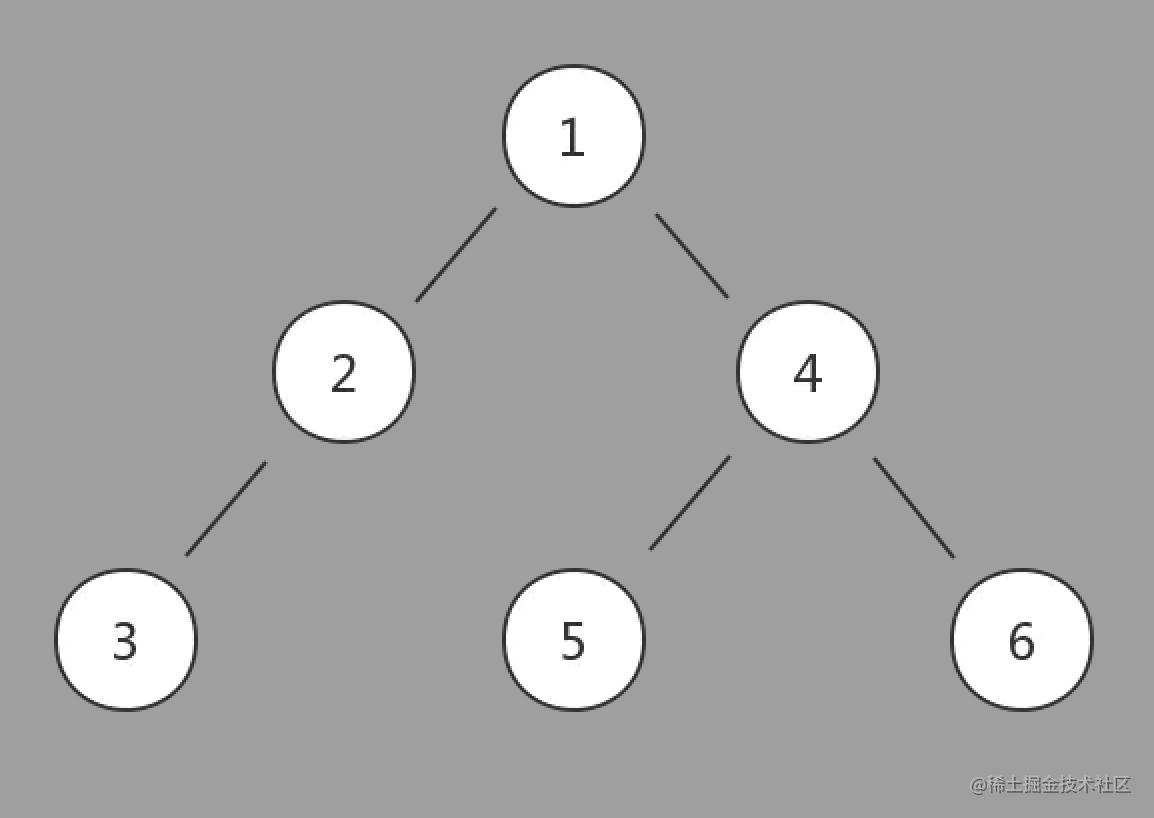# 二叉树遍历（前序、中序、后序）### 1 下面为二叉树的数据结构

``````let root = {
val: 1,
left: {
val: 2,
left: {
val: 3
},
right: {
val: 4
}
},
right: {
val: 5,
}
}• 先序遍历结果(根左右)：1 2 3 4 5 6
• 中序遍历结果(左根右)：3 2 1 5 4 6
• 后序遍历结果(左右根)：3 2 5 6 4 1

• 先序：遍历到一个节点，即可输出该节点的值，并继续遍历左、右子树
• 中序：遍历到一个节点，将其暂存，遍历完左子树后，再输出该节点的值，再遍历右子树
• 后序：遍历到一个节点，将其暂存，遍历完左右子树后，在输出该节点的值

### 2 先序遍历

• 取跟节点为目标节点，开始遍历
• 1.访问目标节点值
• 2.左子树入栈 -> 直至左子树为空的节点
• 3.节点出栈，以右子树为目标节点，再依次执行1、2、3
``````function test2(root) {
let stack = [];
let result = [];
while(root || stack.length > 0) {
while(root) {
result.push(root.val);
stack.push(root);
root = root.left;
}
root = stack.pop();
root = root.right;
}
return result;
}

### 2 中序遍历

• 取跟节点为目标节点，开始遍历
• 1.左子树入栈 -> 直至左子树为空的节点
• 2.节点出栈 -> 访问该节点
• 3.以右子树为目标节点，再依次执行1、2、3
``````function test(root) {
let result = [];
let stack = [];
while(root || stack.length > 0) {
while(root) {
stack.push(root);
root = root.left;
}
root = stack.pop();
result.push(root.val);
root = root.right;
}
return result;
}

### 3 后序遍历

``````function test(root) {
const result = [];
const stack = [];
let last = null;
while (root || stack.length > 0) {
while (root) {
stack.push(root);
root = root.left;
}
root = stack[stack.length - 1];
if (!root.right || root.right === last) {
root = stack.pop();
result.push(root.val);
last = root;
root = null;
} else {
root = root.right;
}
}
return result;
}